## General Chemistry

In a displacement reaction, an ion (or atom) in a compound is replaced by an ion (or atom) of another element. We can use this general scheme for a visual representation of displacement reactions:There are a few main types of displacement reactions, so let’s go over them one by one.

## Hydrogen Displacement

Some metals are capable of displacing hydrogen (H+) in the form of H2 gas from water and acids. The reactions where hydrogen gas is released by displacing the H+ ions are called hydrogen displacement reactions.

For example, if we react zinc with hydrochloric acid, the hydrogen is displaced in the form of H2 gas:

Zn(s) + 2HCl(aq) → ZnCl2(aq) + H2(g)

Some of the alkali and alkali earth metals can also displace hydrogen from water.

2Na(s) + 2H2O(l) → 2NaOH(aq) + H2(g)

Replacing hydrogen from water is more difficult and there are fewer metals capable of doing it.

To predict whether a metal will be able to displace hydrogen, the activity series (electrochemical series) chart is used.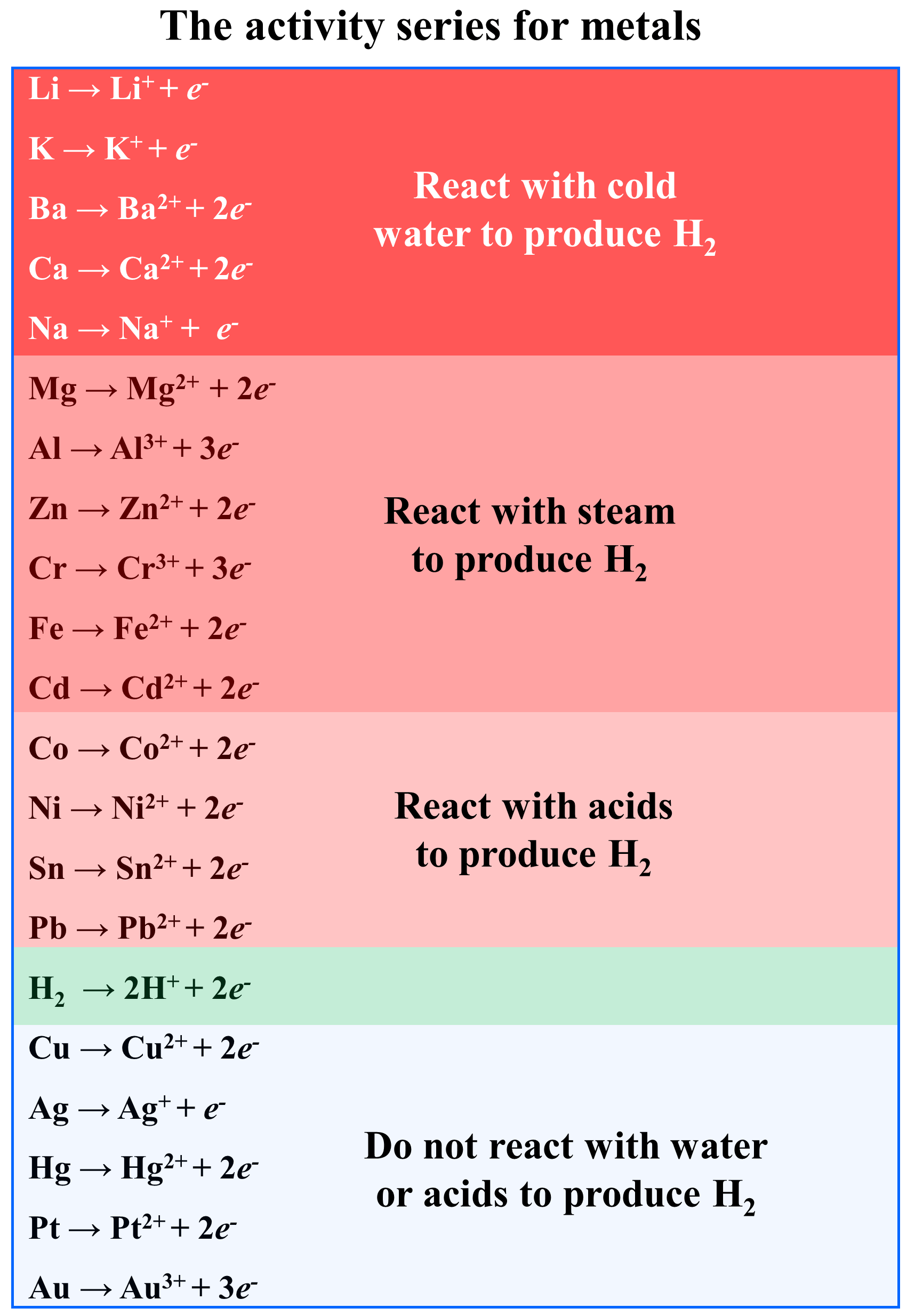All you need to remember for this is that any metal above hydrogen will displace it from water. The ones that stand below hydrogen, cannot displace it from water and therefore, they won’t react with water.

Li(s) + H2O(l) → LiOH(aq) + H2(g)

Ag(s)  + H2O(l) → no reaction

This does not, however, mean that metals below hydrogen cannot react with any acid. They will, but not every time the hydrogen will be the product of the reaction. For example, copper reacts with concentrated nitric acid in the following redox reaction:

3Cu(s) + 8HNO3(aq) → 3Cu(NO3)2(aq) + 2NO(g) + 4H2O(l)

So, the strict borderline for the activity of a metal is whether it reacts with water or not.

While Group 1A(1) metals and Ca, Sr, and Ba from Group 2A(2) readily react with water to displace H2, less active metals can still displace H2 from acids. For example, Ni and even Cu are not as reactive of a metal, but they can still displace H2 gas from acidic solutions with high concentrations.

## Metal Displacement Reactions

In metal displacement reactions, a metal in a compound is displaced by another metal that has been reacted in the elemental form. For example, magnesium can displace Co2+ ions from CoCl2 forming MgCl2 salt:

Mg(s)  + CoCl2(aq) → MgCl2(aq)  + Co(s)

The question is how do we know which metal can displace another metal from a salt?

You need to remember, that the activity series is also used to predict whether the given metal can displace another one. And this again will depend on their position in the activity series. If it stands above the metal in the compound, a displacement reaction will occur, while the reverse reaction won’t be possible.

For example, Zn stands above Cu, so zinc metal will displace copper ions from copper salts.

Zn(s) + CuSO4(aq) → ZnSO4(aq) + Cu(s)

The reverse reaction won’t occur unless external energy is applied which we will discuss in electrochemistry.

## Halogen Displacement

Halogens are also capable of displacing another halogen ions in the form of X2 molecules. For example, Cl2 is used to prepare I2 from seawater where iodide ions are present:

Cl2(g)  + 2NaI(aq) → 2NaCl(aq) + I2(s)

This is a redox reaction, where Cl2 is the oxidizing agent and I2 is the reducing agent as it is being reduced. If you have not covered the oxidation state, and redox reaction in general, do not worry about this terminology. What you need to remember is that any halogen standing above the other one in the periodic table, can displace it from a compound.

F2 > Cl2  > Br2 > I2

# Double-Displacement (Metathesis) Reactions

In the examples above, we have seen that one element displaces another one from a compound. Now, when there are two species changing partners, we call double-displacement or metathesis reactions.

The most common examples of double-displacement reactions are the precipitation reactions which occur when the cations and anions of two compounds are combined and an insoluble compound is formed.

For example, when an aqueous solution of sodium chromate (Na2CrO4) is added to an aqueous solution of silver nitrate (AgNO3), a dark orange precipitate of silver chromate (Ag2CrO4) is formed:

Na2CrO4(aq) + AgNO3(aq) → Ag2CrO4(s) + NaNO3(aq)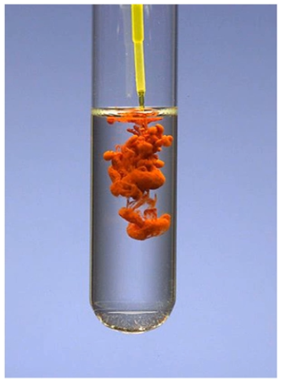To predict the products of a double displacement reaction, you need to know the formulas and the charges of the ions in the starting materials and use the solubility rules to identify the precipitate in the reaction. The solubility of common ions is summarized in the table below: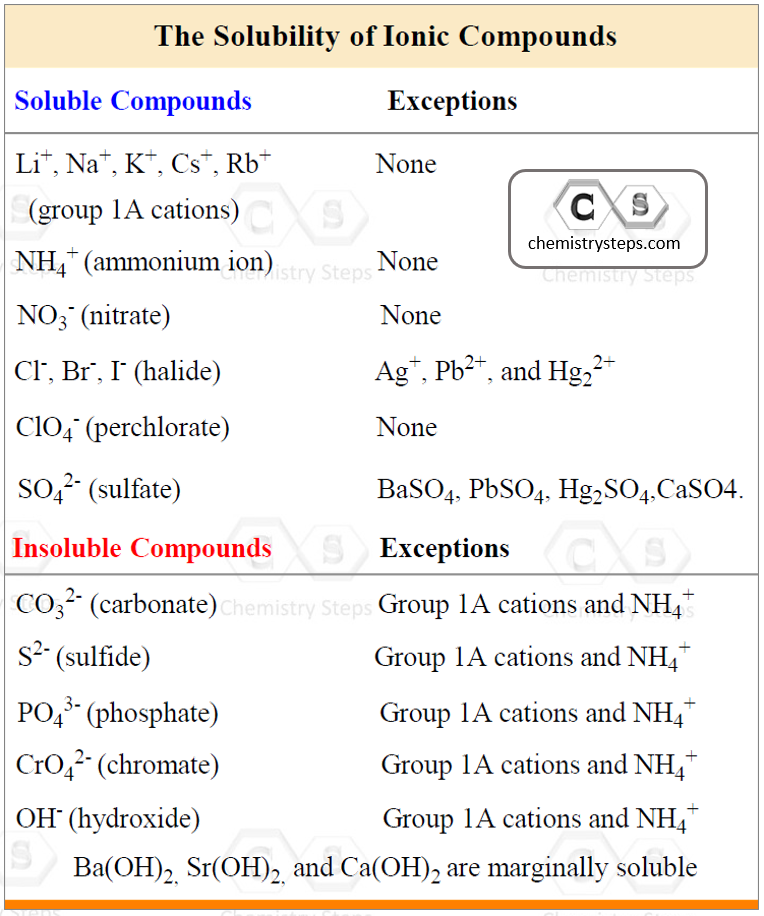For example, let’s determine the products of the reaction between AgNOand CaCl2:

AgNO+ CaCl2 ?

First, we realize that the metals are going to be Ag+ and Ca2+ ions. Now, for the anions, the chlorine forms the Cl ion, and the nitrate has a formula of NO3. Now, this may seem easy (and it should not be very difficult to know the formulas of the ions), but if you have not practiced the names and formulas of common ions, you may get stuck or come up with a wrong formula for the products. Therefore, use the table below to review the formulas of the common polyatomic ions as well.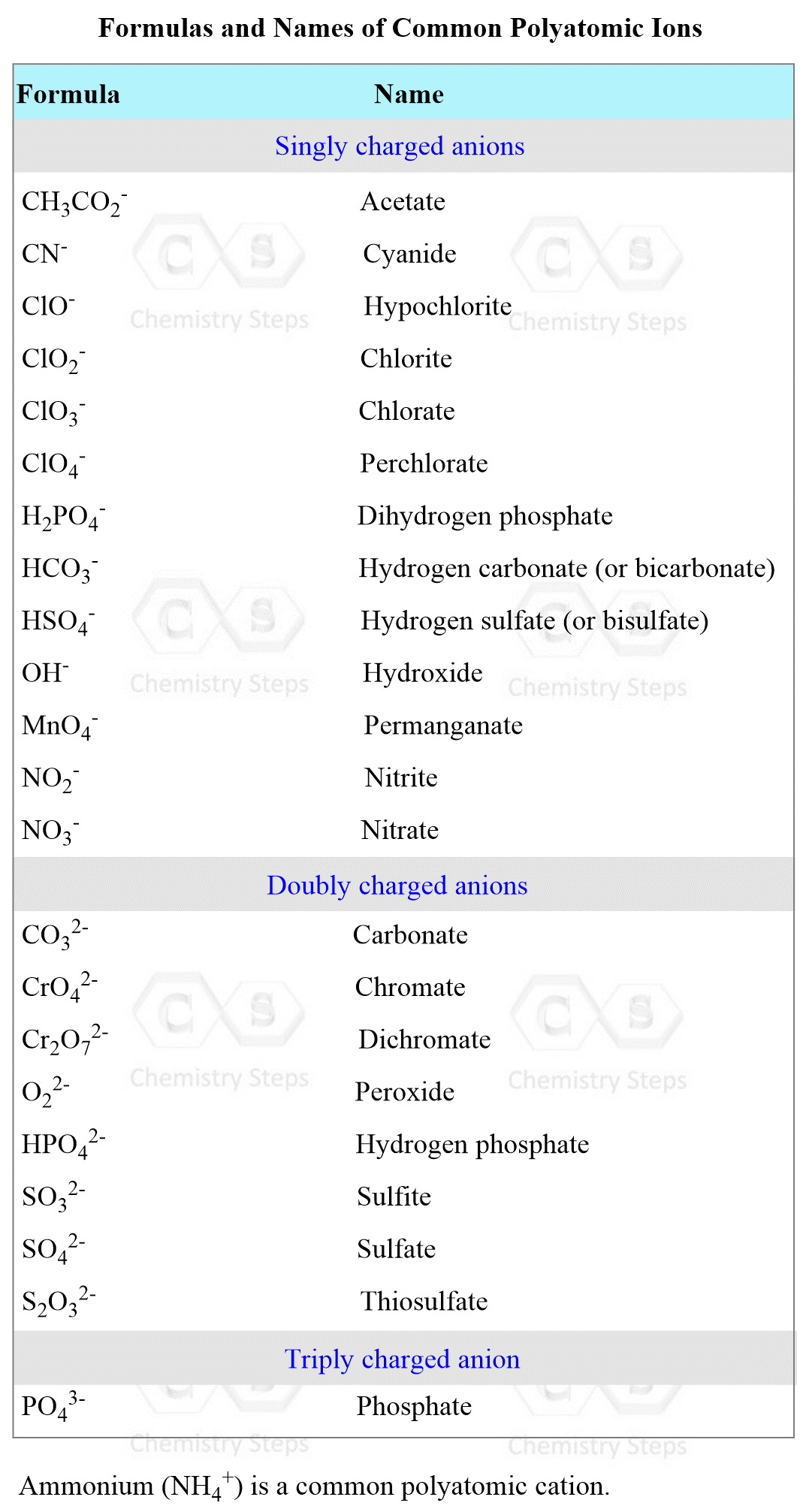Once we know the charges of the ions, here is what we do:

Complete and balance the following equation between solver nitrate and calcium chloride:

AgNO3(aq) + CaCl2(aq) → ?

1) Exchange the cations and anions. Only carry the subscripts that are part of the formula. I.e., we don’t carry the subscript of Cl, but we do for the nitrate since the formulas are Cl and NO3: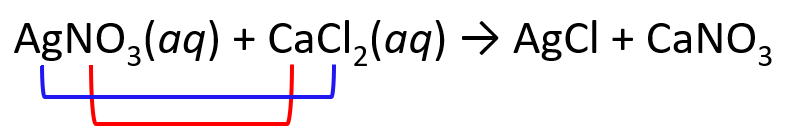2) Place the ionic charges on top of elements and adjust the subscripts: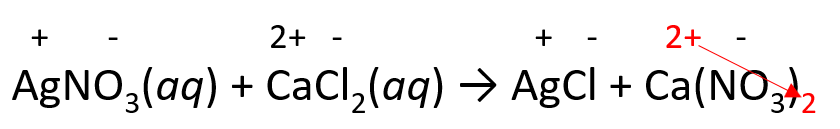3) Balance the equation with coefficients and remove the charges:

2AgNO3(aq) + CaCl2(aq) → 2AgCl + Ca(NO3)2

4) Label the soluble compounds as (aq). Determine the precipitate (in this case AgCl) and label it as (s) – solid.

2AgNO3(aq) + CaCl2(aq) → 2AgCl(s) + Ca(NO3)2(aq)

AgCl is a solid (precipitate) because the salts of halides are soluble except when combined with Ag+, Pb2+, and Hg22+.

# Acid-Base Reactions as Displacement Reactions

The reaction between acids and bases is also an example of a double-displacement reaction. For example, what products are formed when aluminum hydroxide, Al(OH)3 is reacted with sulfuric acid, H2SO4?

Al(OH)3 + H2SO4 ?

Al forms the Al3+ ion, and it is going to combine with the anion of the acid – sulfate ion, SO42-.

To predict the formula of the product, write the ions next to each other and move the charges across to add the correct subscripts: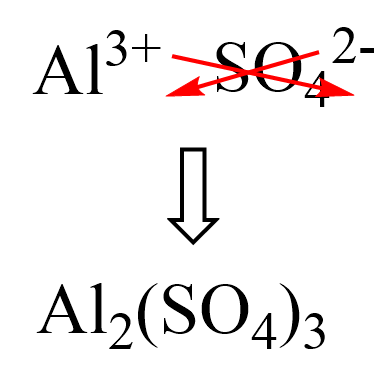The other product of the reaction is water which forms by combining the H+ and OH ions.

Al(OH)3(aq) + H2SO4(aq) → Al2(SO4)3(aq) + H2O(l)

In the end, remember to balance the reaction:

2Al(OH)3(aq) + 3H2SO4(aq) → Al2(SO4)3(aq) + 6H2O(l)

There is no precipitate forming in this reaction, however, water can be considered the driving force of the reaction since it is not an ionic compound. Remember, the reactions in aqueous solutions occur if there is a precipitate, gas, or liquid is formed. Otherwise, it will only be a mixture of ions floating in the solution. For example, if we mix MgCl2 with NaNO3, there will simply be Mg2+, Na+, Cl-, and NO3 ions floating in the solution because of the dissociation of the salts.

Being able to predict the products of a reaction is very important partly because it is required for solving problems on determining the amount of the product based on the stoichiometry of the reaction.

Check Also

#### Practice

1.

Complete and balance each of the following molecular equations in aqueous solution:

a) AgNO3(aq) + CaCl2(aq) →

b) HNO3(aq) + Na2CO3(aq) →

c) K2CO3(s) + HClO3(aq) →

d) Al(OH)3(s) + HBr(aq) →

e) H3PO4(aq) + Fe(OH)3(s) →

f) BaCl2(aq) + K2SO4(aq) →

a)
This content is available to registered users only. Click here to Register! By joining Chemistry Steps, you will gain instant access to the answers and solutions for all the Practice Problems and the powerful set of General Chemistry 1 and 2 Summary Study Guides.
b)
This content is available to registered users only. Click here to Register! By joining Chemistry Steps, you will gain instant access to the answers and solutions for all the Practice Problems and the powerful set of General Chemistry 1 and 2 Summary Study Guides.
c)